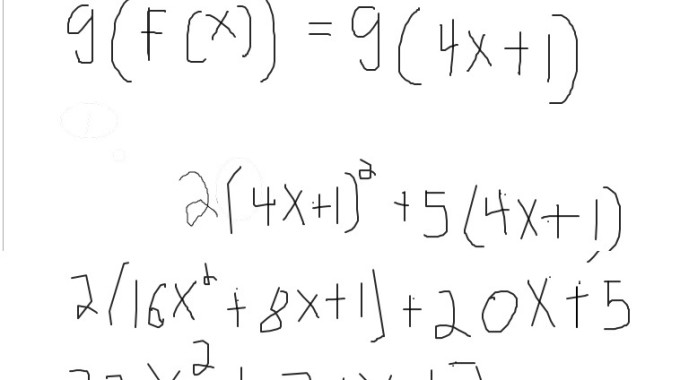Saltear al contenido principal# Funciones compuestasStudent I’m working on composite functions, but do not understand what the «domain» is or how to find it. I can work through the problem, but am lost when it comes to the domain. Please help!

(Tutor) Hi, my name is Juan. How are you today?

Student I’m well. How are you?

(Tutor)  I’m good :D, ok let’s get started!

Student ok. I’m needing to understand, what the «domain» is and how to find it?

(Tutor)  Sure!

the domain is all the values that «x» can take
for example
let’s find the domain of f(x)
f(x)=4x+1
in that case does x have any restriction?
I mean is there an x value that we can’t use?

Student no. I don’t think so

(Tutor) correct
so the domain of that function is all real numbers
for example
this function
is there an x value that we can’t use?

Student 0

(Tutor) You got it!

Student ok

(Tutor)  so the domain of this function is
all real numbers except zero
is it more clear?
or not?
should I try to explain it in a different way?

Student yes.. domain is all values that x can be for the given function

(Tutor)  correct! 😀

Student ok

(Tutor) let’s go back to the problem

Student ok

(Tutor)  in this case
the domain of f(x) is all real numbers

Student I think i’m still slightly confused.

(Tutor) in that function
is there any x value that we can’t use?

Student no, i don’t think so

(Tutor) Correct

Student ok

(Tutor) so the domain is all real numbers

Student yes

(Tutor)  Clue: the domain of a polynomial is always all real numbers
f(x) and g(x) are polynomials

Student ok. i see

(Tutor) so the domain will be all real numbers

Student f(g(x))

(Tutor) correct 😀
but they are asking for gof

Student yes

(Tutor)  ok do you know how to find it?

Student g(4x+1)

(Tutor)  yeah!
then
we have to use g
how would it be?
do you need help?

Student i think i’ve got it. I had trouble with the buttons

(Tutor)  ohhh
what are you trying to do?

Student  2(4x +1)squared + 5(4x+1)

(Tutor)  yeah!

Student  2(16x 2 + 8x + 1) + 20x + 5
32x 2 + 16x + 2 + 20 + 5

(Tutor)  it is 20x
but well done!

Student  equals 32x 2 + 36x +7

(Tutor)  😀
You got it!
And what’s the domain of that function?
I understand what you wrote
but it should be written
like…

Student  right, negative on the left

Student Thank you so much for your help! 🙂

(Tutor)  No problem! It was great to work with you!
Bye

Student  thanks. Bye

Colaborador: Juan Cruz, Tutor Colombiano de Álgebra

Volver arriba